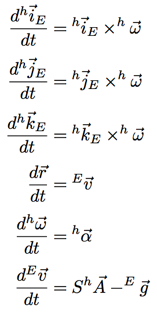# Montagni Project

Motions for Which a Person Feels Upright and Stationary
by Tommaso Montagni

Below are movements for which a person with no visibility feels upright and stationary when in fact they aren’t. Eighteen variables in the differential equations used to model these movements are divided into the 9 variables indicating the initial position on the various planes, 3 variables for the initial orientation, 3 for the initial angular velocity and 3 for the initial linear velocity.By looking carefully at the equations, S, for example, is a 3×3 matrix which converts vector A into Earth-based coordinates. The vector A is the linear acceleration, including gravity, as detected by the human. Then the Earth-based gravitational vector can be subtracted from the vector A to deduce the remaining actual linear acceleration.

The system of equations is constrained to always show a motion where the person, or in fact the “head” that you see, feels upright and stationary no matter the initial conditions. It is worth mentioning that one of the restrictions which is imposed on the system of equations is gravity at 9.81 m/s2

Animation: A Rotating motion in which a person feels upright and stationary.
Description: The initial conditions that generated this particular motion are as follows: an angular velocity of 0,0,10 rad/s in the x,y,z planes respectively and a linear velocity of 0,0,0 m/s in the x,y,z planes respectively. Here, some velocity in the z-axis has been added which makes the head rise up in the air before falling.

Animation: A vertical falling motion in which a person feels upright and stationary.
Description: The initial conditions that generated this particular motion are as follows: an angular velocity of 0,10,0 rad/s in the x,y,z planes respectively and a linear velocity of 0,0,0 m/s in the x,y,z planes respectively. Here, the head is in fact somersaulting such that it feels a continuous push in the upright direction just like having a jetpack. Hence, when the head is tilted upside down, the jetpack is pushing downwards creating the same feeling as having gravity act upon it and then as it rotates in the upright direction the head slows its downwards falling motion, just like one can expect from a jetpack.

Animation: A horizontal falling motion in which a person feels upright and stationary.
Description: The initial conditions that generated this particular motion are as follows: an angular velocity of 0,10,10 rad/s in the x,y,z planes respectively and a linear velocity of 10,10,10 m/s in the x,y,z planes respectively.

Animation: A curved rising and falling motion in which a person feels upright and stationary.
Description: The initial conditions that generated this particular motion are as follows: an angular velocity of 0,2,2 rad/s in the x,y,z planes respectively and a linear velocity of 0,0,20 m/s in the x,y,z planes respectively.

Animation: A curved rising and falling motion in which a person feels upright and stationary.
Description: The initial conditions that generated this particular motion are as follows: an angular velocity of 10,0,10 rad/s in the x,y,z planes respectively and a linear velocity of 0,0,20 m/s in the x,y,z planes respectively.

Animation: A horizontal falling motion in which a person feels upright and stationary.
Description: The initial conditions that generated this particular motion are as follows: an angular velocity of 0,0,2 rad/s in the x,y,z planes respectively and a linear velocity of 10,0,0 m/s in the x,y,z planes respectively.

Animation: A curved rising and falling motion in which a person feels upright and stationary.
Description: The initial conditions that generated this particular motion are as follows: an angular velocity of 0,2,2 rad/s in the x,y,z planes respectively and a linear velocity of 0,5,20 m/s in the x,y,z planes respectively.

Animation: A horizontal falling motion in which a person feels upright and stationary.
Description: The initial conditions that generated this particular motion are as follows: an angular velocity of 0,0,20 rad/s in the x,y,z planes respectively and a linear velocity of 20,20,0 m/s in the x,y,z planes respectively.

Animation: A horizontal falling motion in which a person feels upright and stationary.
Description: The initial conditions that generated this particular motion are as follows: an angular velocity of 0,2,8 rad/s in the x,y,z planes respectively and a linear velocity of 0,20,0 m/s in the x,y,z planes respectively.

Animation: A curved rising and falling motion in which a person feels upright and stationary.
Description: The initial conditions that generated this particular motion are as follows: an angular velocity of 0,2,8 rad/s in the x,y,z planes respectively and a linear velocity of 0,5,5 m/s in the x,y,z planes respectively.

Animation: A horizontal falling motion in which a person feels upright and stationary.
Description: The initial conditions that generated this particular motion are as follows: an angular velocity of 5,0,8 rad/s in the x,y,z planes respectively and a linear velocity of 8,0,5 m/s in the x,y,z planes respectively.

Extension of the project

There are many ways in which this project can be developed. Examples of these are in situations of no gravity, where people feel as if a direction is upwards, but two different people may not agree. These are all variables, which depend on the visual surround and if he or she is holding or perhaps touching something.

Another example can be applied to the disorientation, which astronauts feel when moving from one module to another, as they are oriented differently. In fact, even here people don’t seem to agree with general trends since some feel upright no matter what they see and others rely heavily on their sight, which can be very misleading. Overall, different people have much different tendencies on what and how they perceive the upright and stationary position, which just helps to enhance the importance of this project.